# ConcurrentHashMap实现原理

ConcurrentHashMap是Java1.5中引用的一个线程安全的支持高并发的HashMap集合类。这篇文章总结了ConcurrentHashMap的内部实现原理，是对于自己理解后的一些整理。

#### 1.HashTable与ConcurrentHashMap的对比

HashTable本身是线程安全的，写过Java程序的都知道通过加Synchronized关键字实现线程安全，这样对整张表加锁实现同步的一个缺陷就在于使程序的效率变得很低。这就是为什么Java中会在1.5后引入ConcurrentHashMap的原因。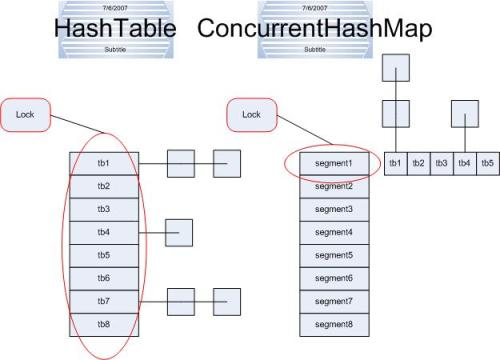#### 2.ConcurrentHashMap的内部结构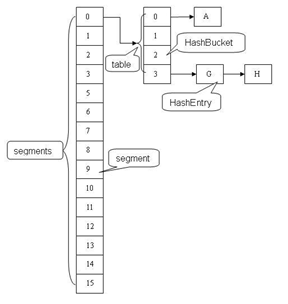ConcurrentHashMap主要有三大结构：整个Hash表，segment（段），HashEntry（节点）。每个segment就相当于一个HashTable。

##### （1）HashEntry类

static final class HashEntry<K,V> {
final K key;                 // 声明 key 为 final 型
final int hash;              // 声明 hash 值为 final 型
volatile V value;           // 声明 value 为 volatile 型
final HashEntry<K,V> next;  // 声明 next 为 final 型

HashEntry(K key, int hash, HashEntry<K,V> next, V value)  {
this.key = key;
this.hash = hash;
this.next = next;
this.value = value;
}
}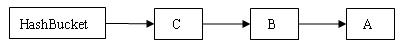##### （2）segment类

Segment 类继承于 ReentrantLock 类，从而使得 Segment 对象能充当锁的角色。每个 Segment 对象用来守护其（成员对象 table 中）包含的若干个桶。

table 是一个由 HashEntry 对象组成的数组。table 数组的每一个数组成员就是散列映射表的一个桶。

count 变量是一个计数器，它表示每个 Segment 对象管理的 table 数组（若干个 HashEntry 组成的链表）包含的 HashEntry 对象的个数。每一个 Segment 对象都有一个 count 对象来表示本 Segment 中包含的 HashEntry 对象的总数。注意，之所以在每个 Segment 对象中包含一个计数器，而不是在 ConcurrentHashMap 中使用全局的计数器，是为了避免出现“热点域”而影响 ConcurrentHashMap 的并发性。

static final class Segment<K,V> extends ReentrantLock implements Serializable {
private static final long serialVersionUID = 2249069246763182397L;
/**
* 在本 segment 范围内，包含的 HashEntry 元素的个数
* 该变量被声明为 volatile 型,保证每次读取到最新的数据
*/
transient volatile int count;

/**
*table 被更新的次数
*/
transient int modCount;

/**
* 当 table 中包含的 HashEntry 元素的个数超过本变量值时，触发 table 的再散列
*/
transient int threshold;

/**
* table 是由 HashEntry 对象组成的数组
* 如果散列时发生碰撞，碰撞的 HashEntry 对象就以链表的形式链接成一个链表
* table 数组的数组成员代表散列映射表的一个桶
* 每个 table 守护整个 ConcurrentHashMap 包含桶总数的一部分
* 如果并发级别为 16，table 则守护 ConcurrentHashMap 包含的桶总数的 1/16
*/
transient volatile HashEntry<K,V>[] table;

/**
* 装载因子
*/
}
##### ConcurrentHashMap 类

 public class ConcurrentHashMap<K, V> extends AbstractMap<K, V>
implements ConcurrentMap<K, V>, Serializable {
/**
* segments 的掩码值
* key 的散列码的高位用来选择具体的 segment
*/

/**
* 偏移量
*/
final int segmentShift;

/**
* 由 Segment 对象组成的数组，每个都是一个特别的Hash Table
*/
final Segment<K,V>[] segments;
}

#### 3.用分离锁实现多个线程间的并发写操作

##### （1）Put方法的实现
public V put(K key, V value) {
if (value == null)  //ConcurrentHashMap 中不允许用 null 作为映射值
throw new NullPointerException();
int hash = hash(key.hashCode()); //计算键对应的散列码

//根据散列码找到对应的 Segment
return segmentFor(hash).put(key, hash, value, false);
}

V put(K key, int hash, V value, boolean onlyIfAbsent) {
lock();    //当前的segment加锁
try {
int c = count;
if (c++ > threshold) //如果超过再散列的阈值
rehash(); //执行再散列，table 数组的长度将扩充一倍
HashEntry<K,V>[] tab = table;

//把散列码值与 table 数组的长度减 1 的值相“与”
//得到该散列码对应的 table 数组的下标值
int index = hash & (tab.length - 1);

//找到散列码对应的具体的那个桶
HashEntry<K,V> first = tab[index];
HashEntry<K,V> e = first;
while (e != null && (e.hash != hash || !key.equals(e.key)))
e = e.next;

V oldValue;
if (e != null) { //如果键/值对以经存在
oldValue = e.value;
if (!onlyIfAbsent)
e.value = value; // 设置 value 值
}
else {  //键/值对不存在
oldValue = null;
++modCount; //添加新节点到链表中，modCont 要加 1

// 创建新节点，并添加到链表的头部
tab[index] = new HashEntry<K,V>(key, hash, first, value);
count = c; //写 count 变量
}
return oldValue;
} finally {
unlock(); //解锁
}
}  

##### （2）Get方法的实现
V get(Object key, int hash) {
if(count != 0) {       // 首先读 count 变量
HashEntry<K,V> e = getFirst(hash);
while(e != null) {
if(e.hash == hash && key.equals(e.key)) {
V v = e.value;
if(v != null)
return v;
// 如果读到 value 域为 null，说明发生了重排序，加锁后重新读取
}
e = e.next;
}
}
return null;
}

ConcurrentHashMap中的读方法不需要加锁，所有的修改操作在进行结构修改时都会在最后一步写count 变量，通过这种机制保证get操作能够得到几乎最新的结构更新。

##### （3）Remove方法的实现
V remove(Object key, int hash, Object value) {
lock(); //加锁
try{
int c = count - 1;
HashEntry<K,V>[] tab = table;
//根据散列码找到 table 的下标值
int index = hash & (tab.length - 1);
//找到散列码对应的那个桶
HashEntry<K,V> first = tab[index];
HashEntry<K,V> e = first;
while(e != null&& (e.hash != hash || !key.equals(e.key)))
e = e.next;

V oldValue = null;
if(e != null) {
V v = e.value;
if(value == null|| value.equals(v)) { //找到要删除的节点
oldValue = v;
++modCount;
//所有处于待删除节点之后的节点原样保留在链表中
//所有处于待删除节点之前的节点被克隆到新链表中
HashEntry<K,V> newFirst = e.next;// 待删节点的后继结点
for(HashEntry<K,V> p = first; p != e; p = p.next)
newFirst = new HashEntry<K,V>(p.key, p.hash,
newFirst, p.value);
//把桶链接到新的头结点
//新的头结点是原链表中，删除节点之前的那个节点
tab[index] = newFirst;
count = c;      //写 count 变量
}
}
return oldValue;
} finally{
unlock(); //解锁
}
}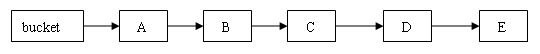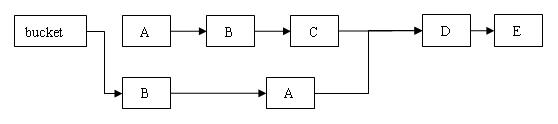##### （4）containsKey方法的实现

containsKey方法操作相对简单，因为它不需要读取值。

boolean containsKey(Object key, int hash) {
if (count != 0) { // read-volatile
HashEntry<K,V> e = getFirst(hash);
while (e != null) {
if (e.hash == hash && key.equals(e.key))
return true;
e = e.next;
}
}
return false;
} 

#### 4.总结

• 减小请求同一个锁的频率。
• 减少持有锁的时间。

ConcurrentHashMap 的高并发性主要来自于三个方面：

• 用分离锁实现多个线程间的更深层次的共享访问。
• 用 HashEntery 对象的不变性来降低执行读操作的线程在遍历链表期间对加锁的需求。
• 通过对同一个 Volatile 变量的写 / 读访问，协调不同线程间读 / 写操作的内存可见性。

#### 5.感谢

Java集合—ConcurrentHashMap原理分析